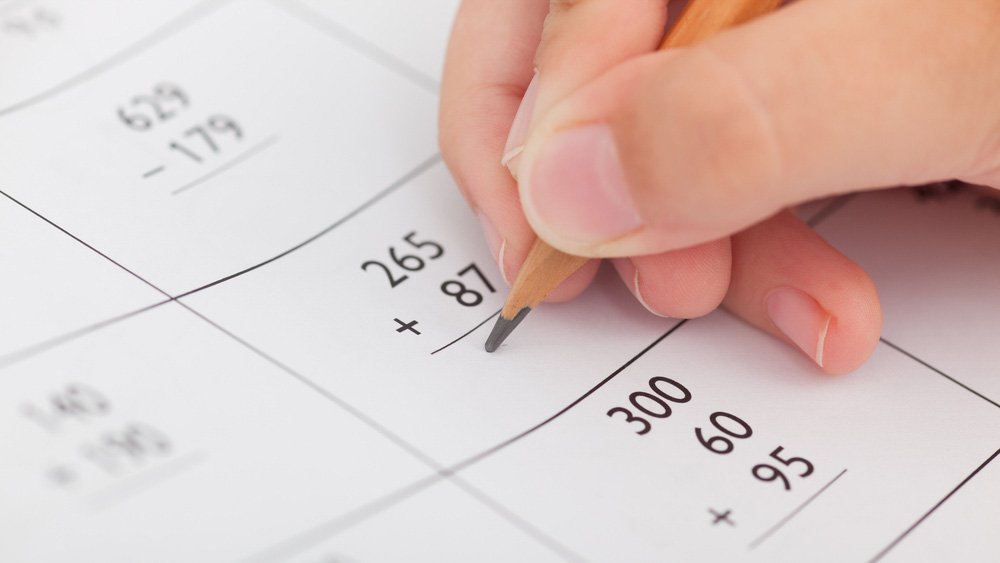# Maths Pre-GCSE

Course Level

Start Date

### Wed 6 Sep 2023

FREE#### Course content

• Whole numbers, fractions, decimals and percentages. Problem solving using mathematical terminology.
• Common measures of time, shape, weight and volume.
• Problem solving using mathematical terminology.
• Handling data and interpreting charts, graphs and maps.
• Statistical measures: mean, mode, median and range.
• Probability.
• Whole numbers, fractions, decimals and percentages. Problem solving using mathematical terminology.
• Common measures of time, shape, weight and volume.
• Problem solving using mathematical terminology.
• Handling data and interpreting charts, graphs and maps.
• Statistical measures: mean, mode, median and range.
• Probability.
• Whole numbers, fractions, decimals and percentages. Problem solving using mathematical terminology.
• Common measures of time, shape, weight and volume.
• Problem solving using mathematical terminology.
• Handling data and interpreting charts, graphs and maps.
• Statistical measures: mean, mode, median and range.
• Probability.

#### Description

This course is designed to help you to develop your numeracy skills and apply them in real life situations, including the world of work, and you will also develop the study skills you need to succeed in your further studies. Students that pass Functional Skills Maths Level 2 before February can transfer to GCSE in the same academic year. Learners who pass Level 2 later in the year are automatically offered a place on GCSE the following academic year.

#### Course content:

• Understand and use whole numbers and understand negative numbers in practical contexts
• Add, subtract, multiply and divide whole numbers using a range of strategies
• Understand and use equivalences between common fractions, decimals and percentages
• Add and subtract decimals up to two decimal places
• Solve simple problems involving ratio, where one number is a multiple of the other
• Use simple formulae expressed in words for one or two-step operations
• Solve problems requiring calculation, with common measures, including money, time, length, weight, capacity and temperature
• Convert units of measure in the same system
• Work out areas and perimeters in practical situations
• Construct geometric diagrams, models and shapes
• Extract and interpret information from tables, diagrams, charts and graphs
• Collect and record discrete data and organise and represent information in different ways
• Find mean and range
• Use data to assess the likelihood of an outcome.

#### Requirements

• This course is open to any learner looking to improve their Maths and gain a stepping stone qualification to GCSE.

All learners no matter what their prior qualifications are will need to be assessed prior to enrollment. The assessments will be booked for you when you contact us.

#### What do I need to do this course?

• 30cm ruler graduated in centimetres and millimetres
• protractor
• pair of compasses
• 2 x black biro pens
• A selection of coloured pens for self-marking
• Highlighters to traffic-light topics
• 2 x sharp HB pencils
• Eraser
• A Casio fx-83GT PLUS Scientific Calculator
• A course file with notepad to keep all your work and revision notes in

#### Teaching Methods

• The lesson is spilt into three parts. The first 50 minutes consists of formal teaching of the more difficult topics within the syllabus. The second 50 minutes consists of using past exam materials. The third 50 minutes is for support with self-directed study tasks.

#### How is your progress checked?

This will be closely monitored in a variety of different contexts including input in class, homework and assessments throughout the course.

#### Extra study or practice required at home.

Learners are expected to review their weekly notes and use MathsWatch to secure their learning. Learners are also expected to spend time working on past assessments.

Grade 1 and 2 topics are mainly self-study but opportunities are given in class to support any misconceptions. Learners are expected to spend up to 2.5 hours a week on self-study at home depending on how quickly they want to pass.

#### What can I do after this course?

After passing their target level exam learners may continue to stay for the whole of the academic year to work towards a higher level. Alternatively they can rejoin the class the following academic year. Learners wanting to progress to GCSE will need to have passed Level 2.

FREE

#### Course details

• Tutor: TBC
• Time: 1300-1530
• Provision: FE
• Term: Full year
• Location: Sports Centre
• Full fee: Free
• Concessionary fee: Free

Share: